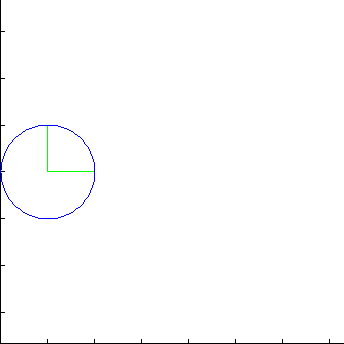Algebra
 Course Homepages: Algebra 1 - R.C.C. Math 95 Intermediate Algebra

### Trigonometry

This graphic below shows the relation between the unit circle and the value of the sine function. The y-value of a point intercepted by a central angle on the unit circle is the y-value of the sine function for that angle.